# Introduction

This presentation will about Linear Regression and it’s alternatives like partial least squares and Penalized regression models. I will go over each one in detail starting with

• Linear Regression
• Penalized Regression Models (LASSO, Ridge Regression and elastic net)

# Linear Regression

• Linear Regression is one of the most basic tools a Data Scientist or Analyst can use for analyzing data. It is also used as a prerequiste for other advanced regression and machine learning techniques.

• In its general form it is the formula

\begin{align} y_i = b_0 +b_1x_{i1} + b_2x_{i2} + ... + b_px_{iP} + \epsilon_i \tag{1} \end{align}

where

• $$y_i$$ represents the numerical response of observation i
• $$b_0$$ represents the estimated intercept
• $$b_j$$ is the estimated coefficient of the jth predictor
• $$x_{ij}$$ is the value of the jth feature of observation i
• P is the number of predictors or explanatory variables
• $$\epsilon_i$$ is random error that can’t be explained by the model

# Ordinary Least Squares

• When performing linear regression, the goal is to find coefficients that minimize the sum-of-squared errors (SSE) between the actual value of the response and the predicted response.

• The (SSE) is defined as the following

\begin{align} SSE = \sum_{i=1}^n (y_i - \hat{y_i})^2 \tag{1} \end{align}

• This equation contains the coefficient estimates for each predictor in a vector.

• It can be shown that the coefficients that minimize the SSE is the matrix equation (Note this would involve a combination of Matrix Algebra and Solving a system of partial derivatives to derive). There is a problem with this solution which will be addressed later in the presentation.

\begin{align} (X^TX)^{-1}X^Ty \end{align}

# Multiple Linear Regression Caveats

• Several flaws in multiple linear regression. For example, the response variable is a linear model and that if the data have some non-linear structure in the mix, the multiple linear regression technique may not be accurate.

• There are many statistics that can tell if the multiple linear regression model you are using is a good one or not.

• Having data with large residuals when doing a multiple regression model can negatively impact your model.

• If the data are shown to not have near constant variance, this is also an indictor that the model needs to be adjusted and may need transformations, feature extraction etc.

• If the residual plot does not follow a normal or near-normal distribution, that is also a bad sign.

• Using Q-Q plots can also be a good metric to test and see as well.

• If there are more predictors than observations, Eq. (2) cannot be inverted.

• Other metrics like $$R^2$$, $$R^2_{adj}$$, p-value and F-test come in handy as well. R’s lm() function gives you these statistics.

# Table Summary of Metrics When Fitting a Multiple Linear Regression Model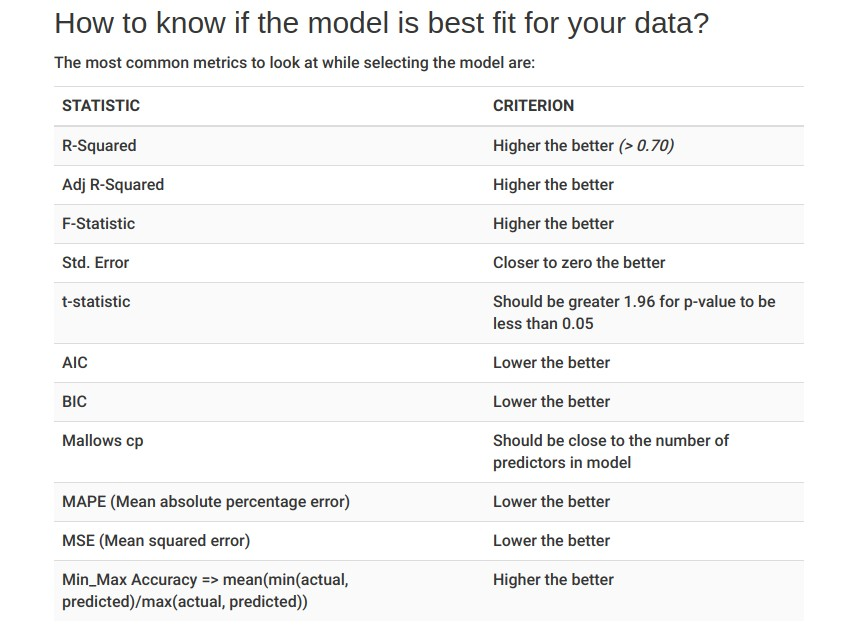# Linear Regression Example: (good fit and not-so-good fit)

• Let’s look at some R code for creating a multiple regression model The R built-in dataset mtcars
# fit a linear model
colnames(mtcars)
##   "mpg"  "cyl"  "disp" "hp"   "drat" "wt"   "qsec" "vs"   "am"   "gear"
##  "carb"
lm_mtcars <- lm(mpg ~ ., data = mtcars)
summary(lm_mtcars)
##
## Call:
## lm(formula = mpg ~ ., data = mtcars)
##
## Residuals:
##     Min      1Q  Median      3Q     Max
## -3.4506 -1.6044 -0.1196  1.2193  4.6271
##
## Coefficients:
##             Estimate Std. Error t value Pr(>|t|)
## (Intercept) 12.30337   18.71788   0.657   0.5181
## cyl         -0.11144    1.04502  -0.107   0.9161
## disp         0.01334    0.01786   0.747   0.4635
## hp          -0.02148    0.02177  -0.987   0.3350
## drat         0.78711    1.63537   0.481   0.6353
## wt          -3.71530    1.89441  -1.961   0.0633 .
## qsec         0.82104    0.73084   1.123   0.2739
## vs           0.31776    2.10451   0.151   0.8814
## am           2.52023    2.05665   1.225   0.2340
## gear         0.65541    1.49326   0.439   0.6652
## carb        -0.19942    0.82875  -0.241   0.8122
## ---
## Signif. codes:  0 '***' 0.001 '**' 0.01 '*' 0.05 '.' 0.1 ' ' 1
##
## Residual standard error: 2.65 on 21 degrees of freedom
## Multiple R-squared:  0.869,  Adjusted R-squared:  0.8066
## F-statistic: 13.93 on 10 and 21 DF,  p-value: 3.793e-07
library(Matrix)
X <- as.matrix(mtcars[,2:11])
y <- as.matrix(mtcarsmpg) solve(t(X) %*% X) %*% (t(X) %*% y) ## [,1] ## cyl 0.35082641 ## disp 0.01354278 ## hp -0.02054767 ## drat 1.24158213 ## wt -3.82613150 ## qsec 1.19139689 ## vs 0.18972068 ## am 2.83222230 ## gear 1.05426253 ## carb -0.26321386 • Note that using the Matrix equation to solve for the coefficients is almost equal to the coefficient values used by R’s lm() function # Summary of Results • We saw from the previous slide that R’s lm() function along with the summmary() function gave us some insight metrics for our model. Let’s review in summary: • Residuals: These values show the summary of the residual values of our fitted model showing the min, 1st quartile, median, 3rd quartile and max value • Coefficients: For each predictor in our model, it shows the estimated coefficient $$b_i$$, the standard error of the coefficient, it’s t-value and it’s p-value. Note that having a low p-value < 0.05 for a 95% confidence interval means that the predictor is significant in the model. We can say we fail to reject the null hypothesis ($$b_i = 0$$) and favor the alternative that is $$b_i \neq 0$$) • Significant codes: These codes show the characters that indicate if the p-value is less than a certain threshold. It’s used more for readbility. • Residual standard error: This is nothing more than the positive squared root of the mean square error that is $$RSE = \sqrt{MSE}$$ • R-Squared and Adjusted R-Squared: The $$R^2$$ and $$R^2_{adj}$$ are the R-squared and Adjusted R-squared values respectively. Mathematically, they are calculated as \begin{aligned} R^2 = 1 - SSE/SST = \sum{(y - \hat{y})^2} / \sum{(y - \bar{y})^2} \\ R^2_{adj} = R^2\big(n-1 / n-k-1 \big) \end{aligned} with $$\hat{y}$$ = estimate of y (from multiple regression model) and $$\bar{y}$$ = mean of y values and n and k are the number of observations and predictions respectively. # Residual Plots • Finally, let’s look at some plots based on our model if it is a good fit in explaining the relationship to mpg (miles per gallon) • Residual Plots (If the residual plot follows a near normal distribution, this is a good sign) hist(lm_mtcarsresiduals)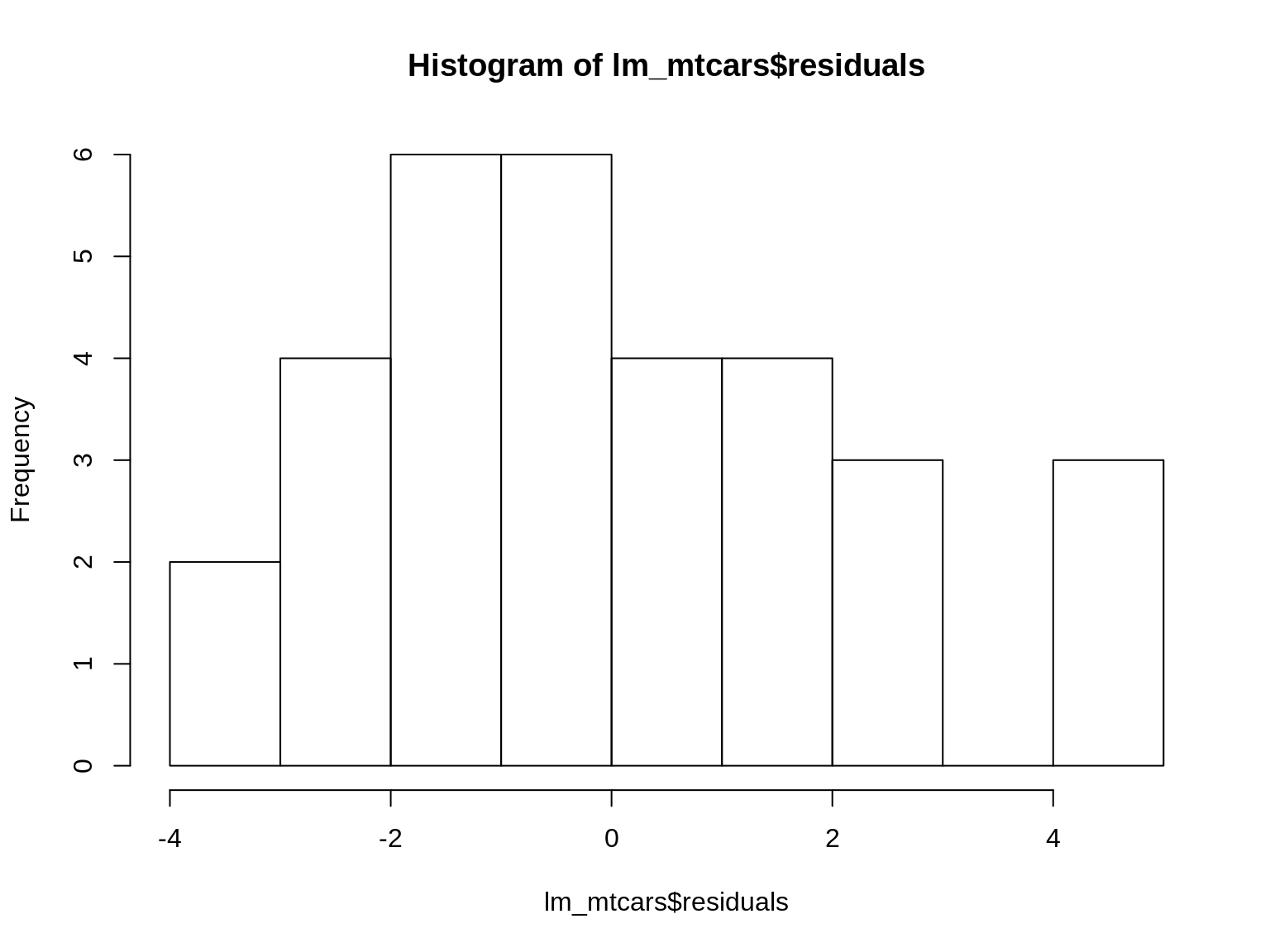• Q-Q plot and REsidual Plot
qqnorm(lm_mtcars$residuals) qqline(lm_mtcars$residuals)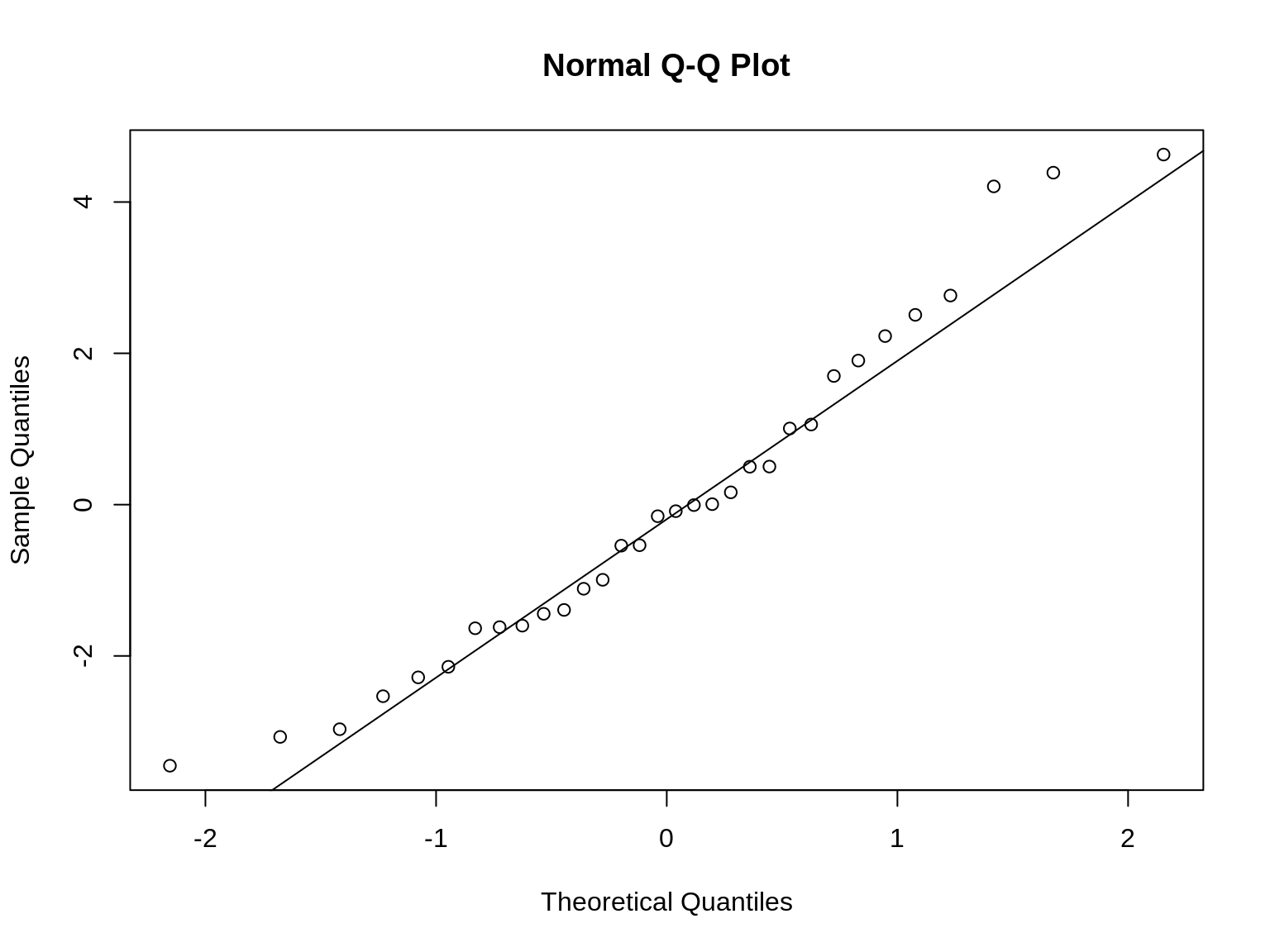plot(mtcars$mpg, lm_mtcars$residuals)
abline(0, 0)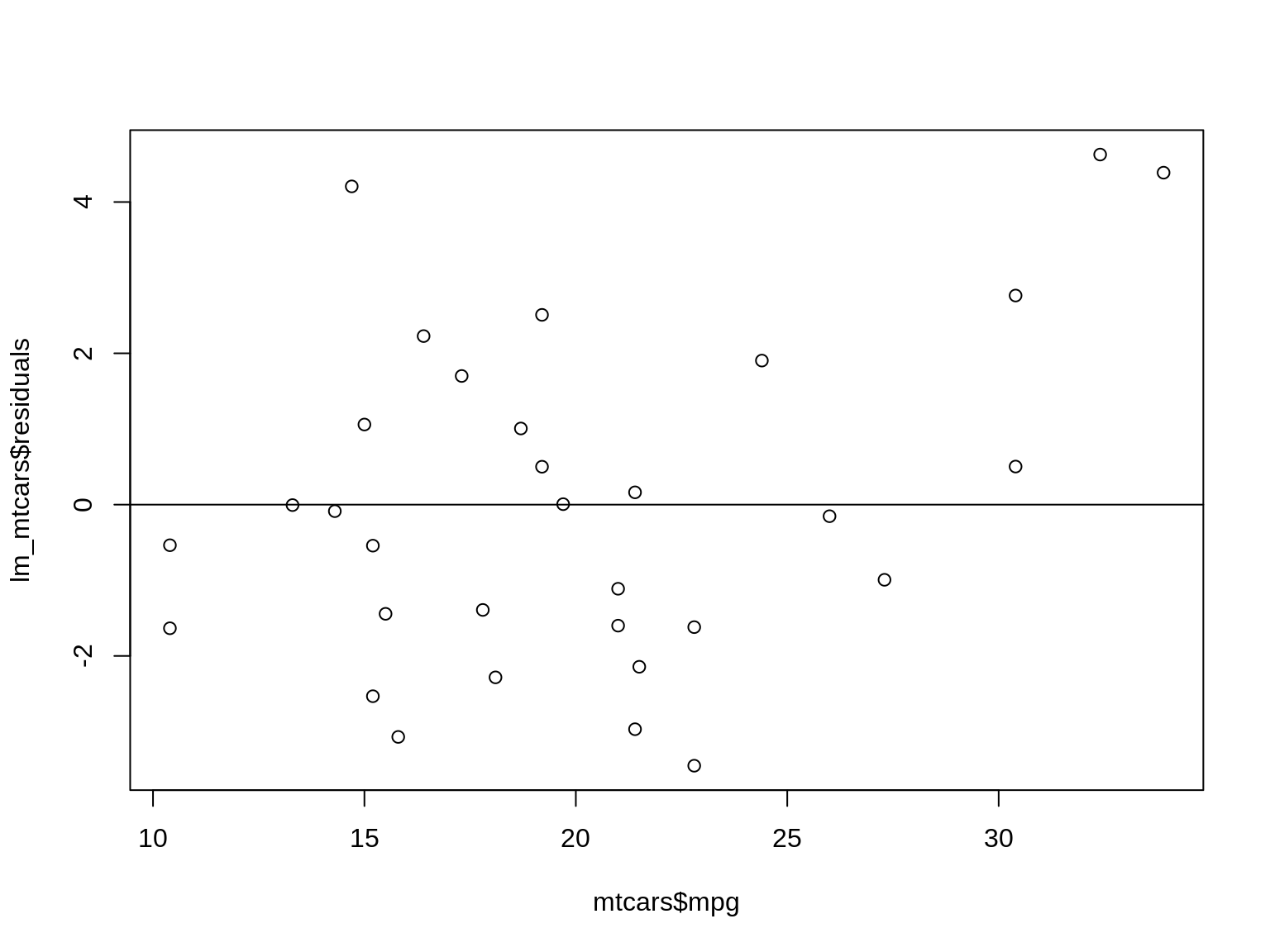# Penalized Models

• Now that we now know about linear regression, let’s introduce the idea of penalized models.

• Sometimes, a model may have overfitting issues and may not be able to accurately predict future observations. Also, penalized models are helpful when there are issues with collinearity.

• Another issue is that if there are large correlations between features that the variance will be very large. As such, penalized methods/models help to reduce variance but introduce bias into the picture.

• I will talk about 3 penalized models in this presentation

• Ridge Regression

• LASSO Regression (Least Absolute shrinkage and selection operator) model

• Elastic net (Ridge Regression and LASSO penalties combined)

• These regularization methods impose penalities on coefficients and R has functions to use these techniques along with finding the optimal value of lambda which we’ll see in the next slide.

# Ridge Regression

• Adds a penalty on the sum of the squared regression parameters and is defined by the formula below:

\begin{aligned} SSE_{L_2} = \sum_{i=1}^n (y_i - \hat{y_i})^2 + \lambda \sum_{j=1}^P \beta_j^2 \end{aligned}

• $$L_2$$ is for the second-order penalty hence the squared value in the parameter estimates.

• What the second term (penalty) does is that the coefficient estimates can become large if there is a reduction on the SSE.

• For large $$\lambda$$ reduces the estimates near 0. However, the parameters never become actually zero and in addition, ridge regression does not perform feature selection.

# Ridge Regression Example

• Let’s use the R library glmnet to build a ridge regression model using the mtcars dataset as an example.
library(glmnet)
## Loading required package: foreach
## Loaded glmnet 2.0-16
library(dplyr)
##
## Attaching package: 'dplyr'
## The following objects are masked from 'package:stats':
##
##     filter, lag
## The following objects are masked from 'package:base':
##
##     intersect, setdiff, setequal, union
# fit a ridge regression model using all features
# alpha = 0 is the ridge regression method alpha =1 is LASSO
# variables X and y are from earlier slides regarding linear regression

# center the response variable mpg
y <- y %>% scale(center = TRUE, scale = FALSE)
ridge_mpg <- glmnet(X, y, alpha = 0, standardize = TRUE)
summary(ridge_mpg)
##           Length Class     Mode
## a0         100   -none-    numeric
## beta      1000   dgCMatrix S4
## df         100   -none-    numeric
## dim          2   -none-    numeric
## lambda     100   -none-    numeric
## dev.ratio  100   -none-    numeric
## nulldev      1   -none-    numeric
## npasses      1   -none-    numeric
## jerr         1   -none-    numeric
## offset       1   -none-    logical
## call         5   -none-    call
## nobs         1   -none-    numeric
• The glmnet() function ran with many lambda values. To find the optimal lambda value, we can use the cv.glmnet() function. We then plot the lambda values and their MSE’s to see which is the $$min(\lambda)$$ using the lambda.min attribute
cv_fit_mpg <- cv.glmnet(X, y, alpha=0, standardize =  TRUE)
plot(cv_fit_mpg)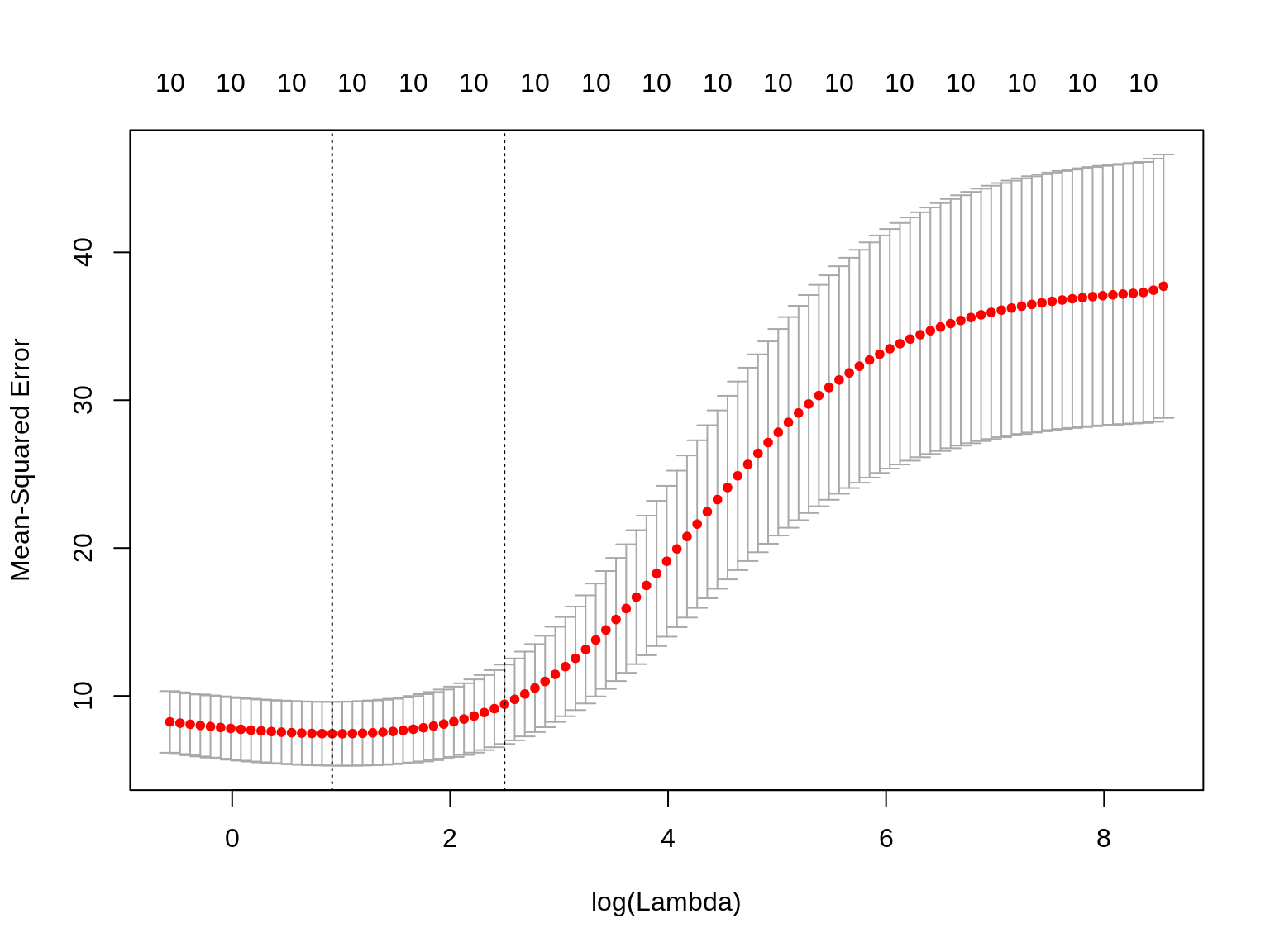• The two lines represent the min $$\lambda$$ value and the 1 standard deviation away from the min $$\lambda$$ value respectively.

• Min $$\lambda$$ for our model:

min_lambda <- cv_fit_mpglambda.min min_lambda ##  2.502772 • With our lambda that minimizes the MSE, let’s see the coefficients as well coef(cv_fit_mpg, s = "lambda.min") ## 11 x 1 sparse Matrix of class "dgCMatrix" ## 1 ## (Intercept) 1.080660889 ## cyl -0.368057153 ## disp -0.005179902 ## hp -0.011713150 ## drat 1.053216800 ## wt -1.264212474 ## qsec 0.164975032 ## vs 0.756163433 ## am 1.655635458 ## gear 0.546651086 ## carb -0.559817881 # LASSO (Least Absolute Shrinkage and Selection Operator) • While ridge regression penalizes the sum of squared coefficnents, LASSO penalizes the sum of absolute values that is: \begin{aligned} SSE_{L_1} = \sum_{i=1}^n (y_i - \hat{y_i})^2 + \lambda \sum_{j=1}^P |{\beta_j}| \end{aligned} • Another neat feature is that LASSO may actually make a coefficient = 0 so therefore it also does feature selection. • Much like Ridge Regression, we can get the $$\lambda$$ value that minimizes the MSE as well as extract the coefficients from it. Note that if a feature coefficient has just ‘.’ is because LASSO penalized that coefficient to be zero and has done feature selection. • In R when using glmnet() and cv.glmnet, set the alpha parameter to be 1 to let R know you’re doing the LASSO technique. • Let’s see an example in the next slide of LASSO in action. # LASSO Example • Using the mtcars dataset, let’s use LASSO to see what are the coefficients and the optimal $$\lambda$$ parameter. # fit a LASSO regression model using all features # alpha = 0 is the ridge regression method alpha = 1 is LASSO # variables X and y are from earlier slides regarding linear regression # center the response variable mpg lasso_mpg <- glmnet(X, y, alpha=1, standardize = TRUE) summary(lasso_mpg) ## Length Class Mode ## a0 79 -none- numeric ## beta 790 dgCMatrix S4 ## df 79 -none- numeric ## dim 2 -none- numeric ## lambda 79 -none- numeric ## dev.ratio 79 -none- numeric ## nulldev 1 -none- numeric ## npasses 1 -none- numeric ## jerr 1 -none- numeric ## offset 1 -none- logical ## call 5 -none- call ## nobs 1 -none- numeric lasso_cv_fit_mpg <- cv.glmnet(X, y, alpha=1, standardize = TRUE) plot(lasso_cv_fit_mpg)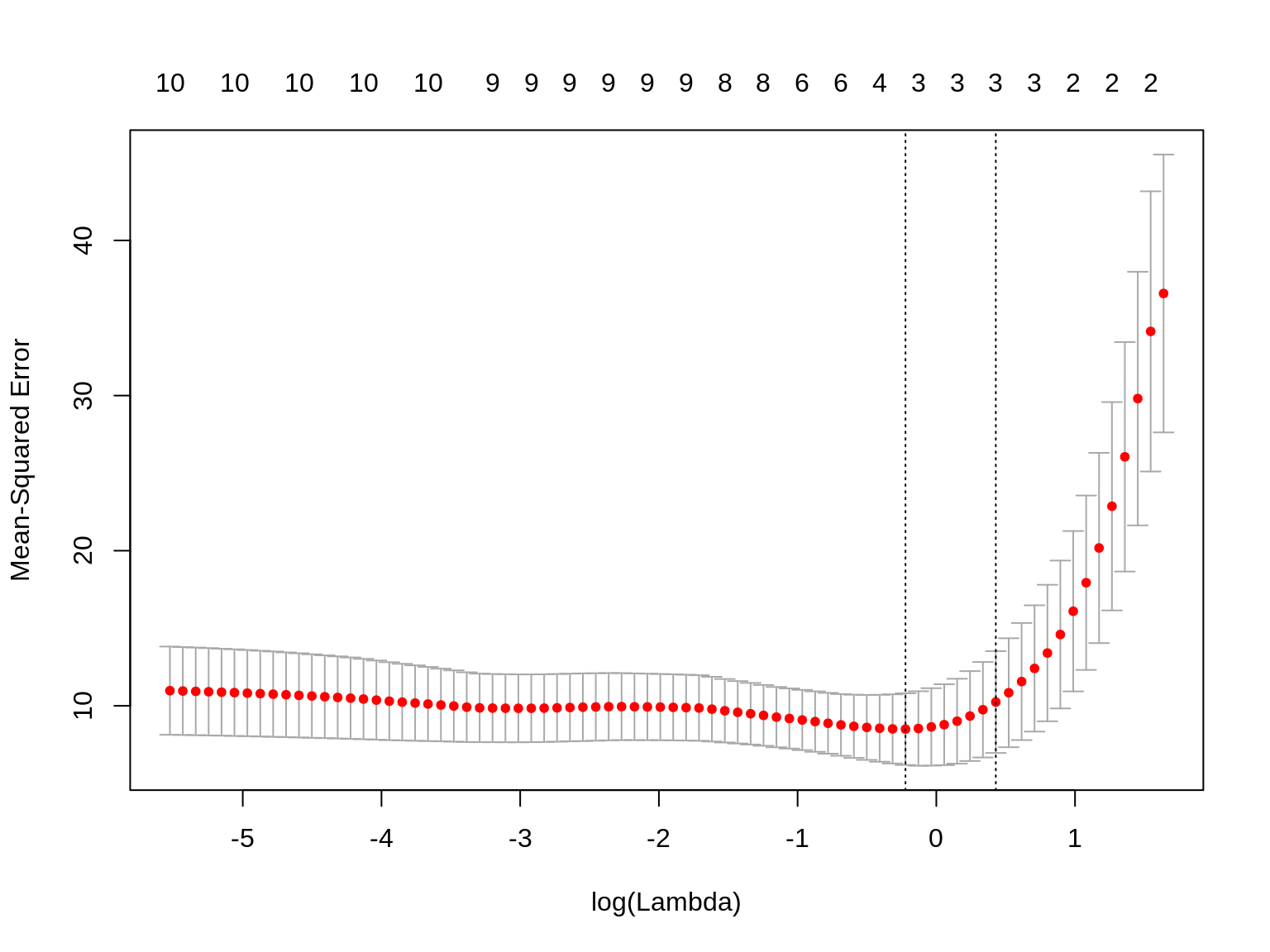• Optimal lambda to minimize MSE lasso_min_lambda <- lasso_cv_fit_mpglambda.min
lasso_min_lambda
##  0.8007036
• Which coefficients were not penalized according to LASSO
coef(lasso_cv_fit_mpg, s = "lambda.min")
## 11 x 1 sparse Matrix of class "dgCMatrix"
##                       1
## (Intercept) 15.90939176
## cyl         -0.88608541
## disp         .
## hp          -0.01168438
## drat         .
## wt          -2.70814703
## qsec         .
## vs           .
## am           .
## gear         .
## carb         .
• Using the “lambda.min” as the lambda value has shown that LASSO only kept 4 variables in our model to use and penalized the rest.

• For either ridge regression or LASSO regression, one can also use “lambda.1se” which means use a lambda value that is 1 standard error from the minimum.

• Using that, you might get a lambda that has a larger or smaller MSE depending on the data.

# Elastic Net (Best of Both Worlds)

• I’ve talked about ridge and LASSO regression. Both are good penalization methods but what if you wanted to use both?

• LASSO does well when there aren’t many parameters and some are already close to 0

• Ridge regressions works well if there are many parameters of almost the same value.

• LASSO may also take away variables that actually may greatly influence the response variable.

• Introducing Elastic Net which combines both and the function is as follows:

\begin{aligned} SSE_{E_{net}} = \sum_{i=1}^n (y_i - \hat{y_i})^2 + \lambda_1 \sum_{j=1}^P \beta_j^2 + \lambda_2 \sum_{j=1}^P |{\beta_j}| \end{aligned}

• With this, you get the feature selection power of LASSO and penalties of both methods in one.

• Elastic Net example

# Elastic Net, use alpha = 0.5

enet_mpg <- glmnet(X, y, alpha=0.5, standardize = TRUE)
summary(lasso_mpg)
##           Length Class     Mode
## a0         79    -none-    numeric
## beta      790    dgCMatrix S4
## df         79    -none-    numeric
## dim         2    -none-    numeric
## lambda     79    -none-    numeric
## dev.ratio  79    -none-    numeric
## nulldev     1    -none-    numeric
## npasses     1    -none-    numeric
## jerr        1    -none-    numeric
## offset      1    -none-    logical
## call        5    -none-    call
## nobs        1    -none-    numeric
enet_cv_fit_mpg <- cv.glmnet(X, y, alpha=0.5, standardize = TRUE)
plot(enet_cv_fit_mpg)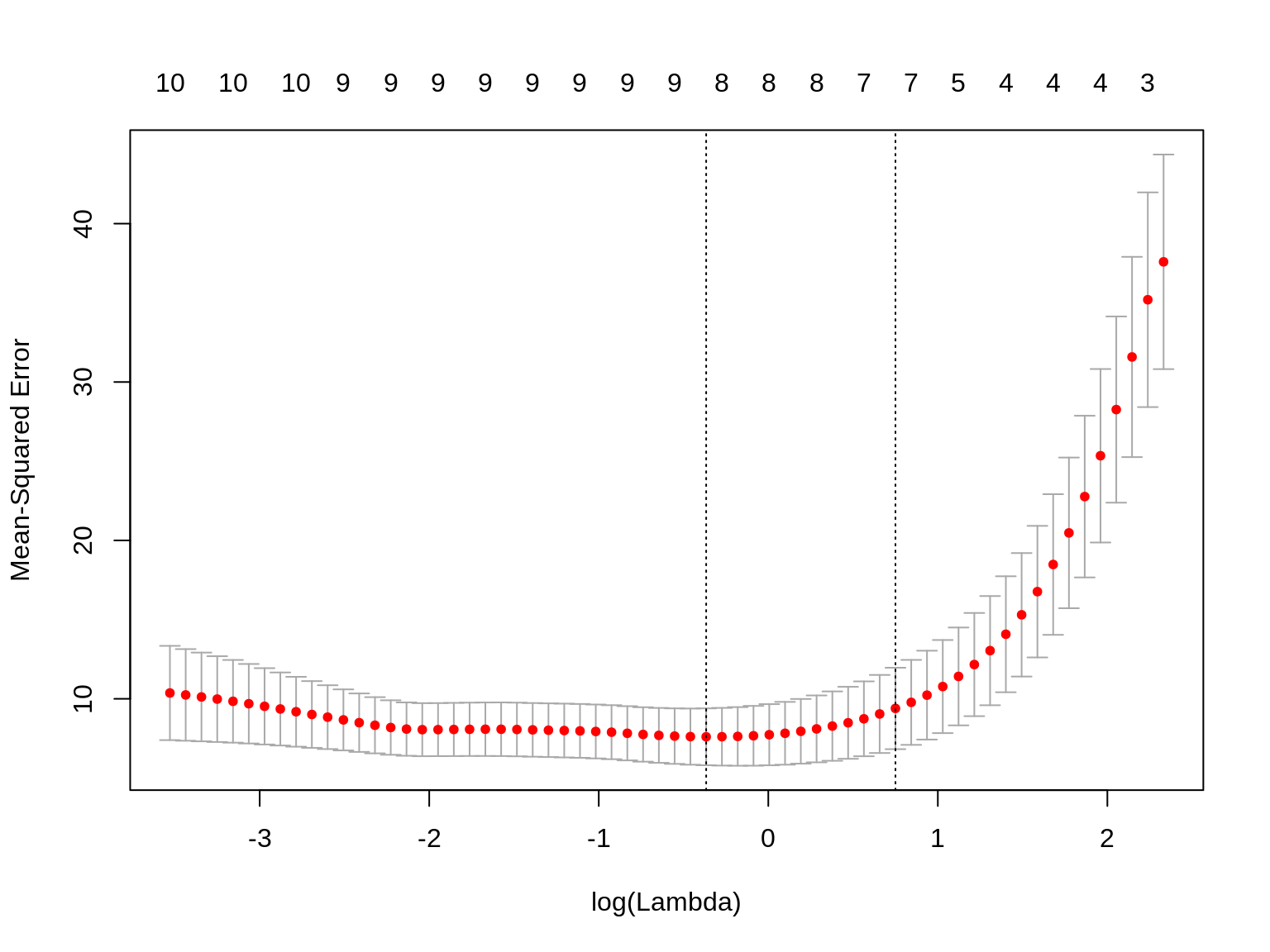enet_min_lambda <- enet_cv_fit_mpg\$lambda.min
enet_min_lambda
##  0.6932109
• Which coefficients were not penalized according to Enet
coef(enet_cv_fit_mpg, s = "lambda.min")
## 11 x 1 sparse Matrix of class "dgCMatrix"
##                        1
## (Intercept) 11.217785623
## cyl         -0.640442781
## disp        -0.000866403
## hp          -0.014738425
## drat         0.624077142
## wt          -2.134845217
## qsec         .
## vs           0.362323658
## am           1.272728484
## gear         .
## carb        -0.335989062

# Summary and Wrap Up

• Linear Regression enables us to create linear models that can minimize the SSE

• There are various ways and diagnostics to see if your linear model is a good fit to your data.

• There is a matrix solution that finds the coefficients of the SSE but may not work if the data exhibit collinearity and/or the number of predictors > number of observations to which the matrix cannot be inverted.

• Penalized methods allow us to decrease the variance while introducing some bias into the mix of our model. This is to also help reduce the MSE of your model.

• Ridge regression, LASSO and elastic net can accomplish this by picking the minimum $$\lambda$$ or 1 standard error (you can also make your own range of $$\lambda$$ values and select the number of folds as well)

• You can select (not shown on the slide) the penalization method by computing each one’s $$R^2$$ value, AIC, BIC.

• You don’t have to select the $$\lambda$$ that minimizes the MSE, you can select your own $$\lambda$$. Experiment with different values and number of folds to tune your model.

Thank you!!!